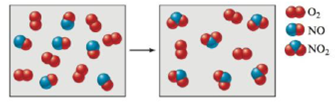Chapter 3, Problem 121E

Chapter
Section
Textbook Problem

Consider the reaction between NO(g) and O2(g) represented below.What is the balanced equation for this reaction, and what is the limiting reactant?

Interpretation Introduction

Interpretation: A balanced chemical equation and the limiting reactant for the given chemical reaction is to be stated.

Concept introduction: According to the law of conservation of mass, mass can neither be created nor destroyed. The mass of reactants is equal to the mass of products formed during a chemical process.

The reactant that is completely consumed during a chemical reaction is termed as the limiting reactant.

To determine: A balanced chemical equation and the limiting reactant for the given chemical reaction.

Explanation

To determine: A balanced chemical equation for the given chemical reaction.

According to the law of conservation of mass, mass can neither be created nor destroyed. The mass of reactants is equal to the mass of products formed. Therefore a chemical equation, having lesser number of moles of an element on either side of a reaction, is balanced using appropriate numerical coefficients to satisfy the law of conservation of mass.

Adding the coefficient 2 to NO and NO2 , balances the number of atoms of nitrogen and oxygen, present on either side of the reaction. The equation thus obtained is,

2NO+O22NO2

To determine: The limiting reactant for the given chemical reaction.

Given

6mol of NO are present on the reactant side.

The stated chemical reaction is,

2NO+O22NO2

2mol of NO react with 1mol of O2

Still sussing out bartleby?

Check out a sample textbook solution.

See a sample solution

The Solution to Your Study Problems

Bartleby provides explanations to thousands of textbook problems written by our experts, many with advanced degrees!

Get Started

Find more solutions based on key concepts